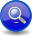#Boost C++ Libraries

...one of the most highly regarded and expertly designed C++ library projects in the world.

This is the documentation for an old version of Boost. Click here to view this page for the latest version.
##### Expectation (```a > b```)
###### Description

Like the Sequence, the expectation operator, ```a > b```, parses two or more operands (`a`, `b`, ... etc.), in sequence:

```a > b > ...
```

However, while the plain Sequence simply returns a no-match (returns `false`) when one of the elements fail, the expectation: `>` operator throws an `expectation_failure``<Iter>` when the second or succeeding operands (all operands except the first) fail to match.

###### Header
```// forwards to <boost/spirit/home/qi/operator/expect.hpp>
#include <boost/spirit/include/qi_expect.hpp>
```

Also, see Include Structure.

###### Model of

Notation

`a`, `b`
`Iter`
###### Expectation Failure

When any operand, except the first, fail to match an `expectation_failure<Iter>` is thrown:

```template <typename Iter>
struct expectation_failure : std::runtime_error
{
Iter first;           // [first, last) iterator pointing
Iter last;            // to the error position in the input.
`info` what_;       // Information about the nature of the error.
};
```
###### Expression Semantics

Semantics of an expression is defined only where it differs from, or is not defined in `NaryParser`.

Expression

Semantics

```a > b```

Match `a` followed by `b`. If `a` fails, no-match. If `b` fails, throw an `expectation_failure<Iter>`

###### Attributes

Expression

Attribute

```a > b```

```a: A, b: B --> (a > b): tuple<A, B>
a: A, b: Unused --> (a > b): A
a: Unused, b: B --> (a > b): B
a: Unused, b: Unused --> (a > b): Unused

a: A, b: A --> (a > b): vector<A>
a: vector<A>, b: A --> (a > b): vector<A>
a: A, b: vector<A> --> (a > b): vector<A>
a: vector<A>, b: vector<A> --> (a > b): vector<A>```

###### Complexity

The overall complexity of the expectation parser is defined by the sum of the complexities of its elements. The complexity of the expectation operator itself is O(N), where N is the number of elements in the sequence.

###### Example
NoteThe test harness for the example(s) below is presented in the Basics Examples section.

Some using declarations:

```using boost::spirit::ascii::char_;
using boost::spirit::qi::expectation_failure;
```

The code below uses an expectation operator to throw an `expectation_failure` with a deliberate parsing error when `"o"` is expected and `"i"` is what is found in the input. The `catch` block prints the information related to the error. Note: This is low level code that demonstrates the bare-metal. Typically, you use an Error Handler to deal with the error.

```try
{
test_parser("xi", char_('x') > char_('o')); // should throw an exception
}
catch (expectation_failure<char const*> const& x)
{
std::cout << "expected: "; print_info(x.what_);
std::cout << "got: \"" << std::string(x.first, x.last) << '"' << std::endl;
}```

The code above will print:

```expected: tag: literal-char, value: o
got: "i"
```

 Copyright © 2001-2010 Joel de Guzman, Hartmut Kaiser Distributed under the Boost Software License, Version 1.0. (See accompanying file LICENSE_1_0.txt or copy at http://www.boost.org/LICENSE_1_0.txt)